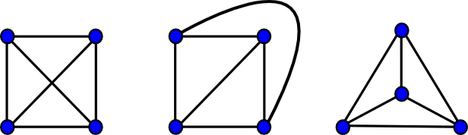# Circuit Breakerd1xlordSUST Intra University Pro...
Limits 1s, 512 MB

In your free time, you like to design various electrical circuits to give a hard time to your local circuit makers by ordering them to build it for you.

Recently, a skilled circuit maker has appeared in town and helping all the others by representing your given circuits as a planar graph. A planar graph is such a graph that can be drawn on the plane in such a way that its edges intersect only at their endpoints. In other words, it can be drawn in such a way that no edges cross each other. It is crucial for a circuit to be a planar graph. You should also note that, even if you draw a graph and think it as non-planar, it maybe possible to represent it as a planar graph. On the image below, by looking at the first image, it may seem like it's a non-planar graph. But it can represented in the following two ways as a planar graph. So previously the circuits you designed thinking as a non-planar graph was somehow represented as a planar graph by this newcomer.Now for tackling this skilled maker, you have to give him a non-planar graph, so that he cannot build a circuit using it. You have already written a computer program that generates a random connected simple graph. Now your work is to determine if the generated graph is non-planar or not.

Checking planarity is hard. After some digging, you came upon these following formula which will ensure that if the conditions are true, the graph will be non-planar. There might be some graphs which are non-planar and doesn't meet these conditions but you don't want to be bothered by them. (The conditions are necessary but not sufficient)

For a connected planar graph having v vertices and e edges, where v ≥ 3, the following holds:

• If there are no cycles of length 3, then e ≤ 2v − 4.
• Else, e ≤ 3v − 6.

Using this formula, you job is to check for each given graph, if it is non-planar or not.

## Input

Input starts with an integer $T$ ($T ≤ 110$), denoting the number of test cases.

Each test case starts with a line containing two integers, $V$ and $E$ ($0 ≤ V ≤ 1000$, $0 ≤ E ≤ 4000$). Each of the next $E$ lines contains two integers, $x$ and $y$, an edge between vertices $x$ and $y$ ($0 ≤ x, y < V$).

## Output

For each test case, print the case number and “Yes” or “No”, without the quotes, depending on the graph being non-planar or not.

## Sample

InputOutput
2
3 3
0 1
0 2
1 2
5 10
0 1
0 2
0 3
0 4
1 2
1 3
1 4
2 3
2 4
3 4

Case 1: No
Case 2: YesuDebug

### Submit

nsucn16.103$Z7nqxEarliest, Oct '16 NorendroFastest, 0.0s NorendroLightest, 131 kB nsucn16.46$03cQQShortest, 594B